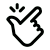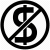# Kai Roofing Calculator

Welcome to our website!

### Here you will find roofing related tools like: roof area calculators, roof materials calculators, pitch calculators, geometric shapes calculator.

All these tools are free and we hope - easy and intuitive to use.

## Roof area calculator (basic )

House Base Area:
/12
Total Roof area: ...

### For a more precise result, please choose a specific shape calculatorWe are looking for collaborators!

## Our principles##### Calculus simplicity!

We are doing lots of calculations in the background in order to ask you for fewer dimensions - we are computing some dimensions based on the ones you provide.

We aim to ask only for the dimensions which are easy to be provided without advanced technical knowledge.##### Uniform roof dimensions!

Because we are trying to make the calculations simpler, we have to assume that your roof dimensions are uniform.

We assume that the mirrored length dimensions and angles are equal so we can only ask for them once. Because of this, the roofing dimensions are ideal.